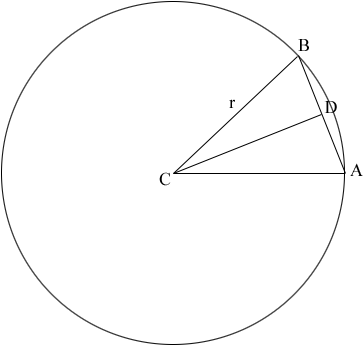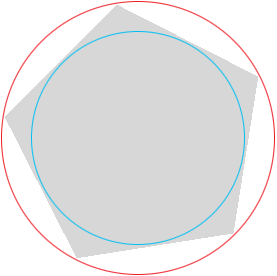SEARCH HOMEMath Central Quandaries & QueriesQuestion from Guy: Like Kay, I also work in stained glass, but in 3-dimensions. I am frequently asked to replicate lamp shades in stained glass where the diameter of the top is different (narrower) from the diameter of the bottom (which is wider). Some people want 5, 6, 7, 8, 10, 12, 16, ,,, n-sided shades. Is there a formula I can use to determine the width of the sides using the angle, if I remember correctly, I think it's called theta. In other words, is there a formula where I can plug in the angle which describes the arc of the circle. For instance, if someone asks for a 7 sided shade, plugging in 51.43 (360/7). I could then use that to determine the width at the top and bottom rings to create the appropriate trapezoids. I've visited a few sites so the formula looks like its a function of sin & cos but they are presented like proofs for teaching. Your site appears to want to actually answer questions without making the inquirer feel stupid.Guy,

Suppose the radius of the base is $r$ units and the lamp is to have $n$ sides. In the diagram below $AB$ is one of the sides of the trapezoid and the measure of the angle $BAC$ is $\large \frac{360}{n}$ degrees.$D$ is the midpoint of $AB$ and hence the measure of the angle $BCD$ is $\large \frac{180}{n}$ degrees. Triangle $BCD$ is a right triangle and hence $\large \frac{|DB|}{r} = cos\left(\frac{180}{n}\right)$ or $|DB| = r cos\left(\frac{180}{n}\right).$ This if $|AB|,$ the length of $AB$ is $L$ then

$L = 2\;r\;cos\left(\frac{180}{n}\right).$

Finally perform the same calculation with $r$ the radius of the top of the lamp.

I hope this helps,
Harley

Guy wrote back with a note that contained

In your diagram, the line AB is a secant, bisecting the circle at 2 points. In my application, the line AB is a tangent intersecting the circle at point D. Therefore r isn't CB but CD. (r is one of the legs of the right triangle, not the hypotenuse.)

Guy,

We did have a miscommunication. In my diagram below, which is the base of a lamp with 5 sides, I took "diameter" to mean the diameter of the red circle where you meant the diameter of the blue circle.Suppose the diameter of this circle is $d$ then in my first diagram $|CD| = d/2.$ Then $\frac{|DB|}{|CD|} = tan\left(\frac{180}{n}\right)$ and hence

$L = d\;tan\left(\frac{180}{n}\right).$

HarleyMath Central is supported by the University of Regina and The Pacific Institute for the Mathematical Sciences.